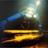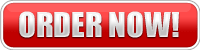# How to i convert the listed gps numbers in this site in degrees, minute, seconds, and .seconds into the format my gps uses degree minutes.mm

ie 44 degrees 17 minutes 13.2612 sec N: 76degrees 0 minutes 15.59988 sec W converted to degrees, minutes.mmm (44 17.xyz)

do you divide the seconds by 60 to convert?

2 Reportshipwreckworld 0  The easiest way to convert the GPS (Degrees °Minutes ' Seconds ") that is displayed in the wreck finder section into Decimal format is with a GPS converter. We have one on the Submit Wreck page here that you can use: http://www.shipwreckworld.com/submit/map/ To answer your question, you would divide Minutes by 60. You would also divide seconds by 3600. Here's the actual C# code for the formula, in case you're a programmer: /// /// Convert (Latitude or Longitude) Degrees, Minutes, Seconds to Decimal (Latitude or Longitude) /// public static double ConvertDmsToDecimal(string degrees, string minutes, string seconds) { // find decimal latitude or longitude double dmsDegrees = degrees == string.Empty ? 0 : Convert.ToDouble(degrees); // empty null value needs to be handled // Set flag if number is negative bool neg = dmsDegrees < 0d; // Work with a positive number, needed for the addition to work right (we add negative sign at the end) dmsDegrees = Math.Abs(dmsDegrees); double dmsMinutes = minutes == string.Empty ? 0 : Convert.ToDouble(minutes) / 60; // empty null value needs to be handled double dmsSeconds = seconds == string.Empty ? 0 : Convert.ToDouble(seconds) / 3600; // empty null value needs to be handled // add values together double ddVal = dmsDegrees + dmsMinutes + dmsSeconds; // make negative if needed ddVal = neg ? (ddVal * -1) : ddVal; // round down a bit ddVal = Math.Round(ddVal, 6, MidpointRounding.AwayFromZero); // Rounds to 6 digits past decimal return ddVal; } You may also use a GPS converter here: http://transition.fcc.gov/mb/audio/bickel/DDDMMSS-...JimKennard 0  Hi Eric, Divide the seconds by 60 and add the result to the minutes: 44deg 17 minutes 13.2612 seconds = 44 deg 17 minutes + 13.2612 / 60 = 44 deg 17.22102 minutes 76 deg 0 minutes 15.59988 / 60 seconds = 76 deg 0.259998 minutes Hope this helps. Jim

## Want to post an answer?

#### Tagged

gps coordinates (1)

#### Related Questions